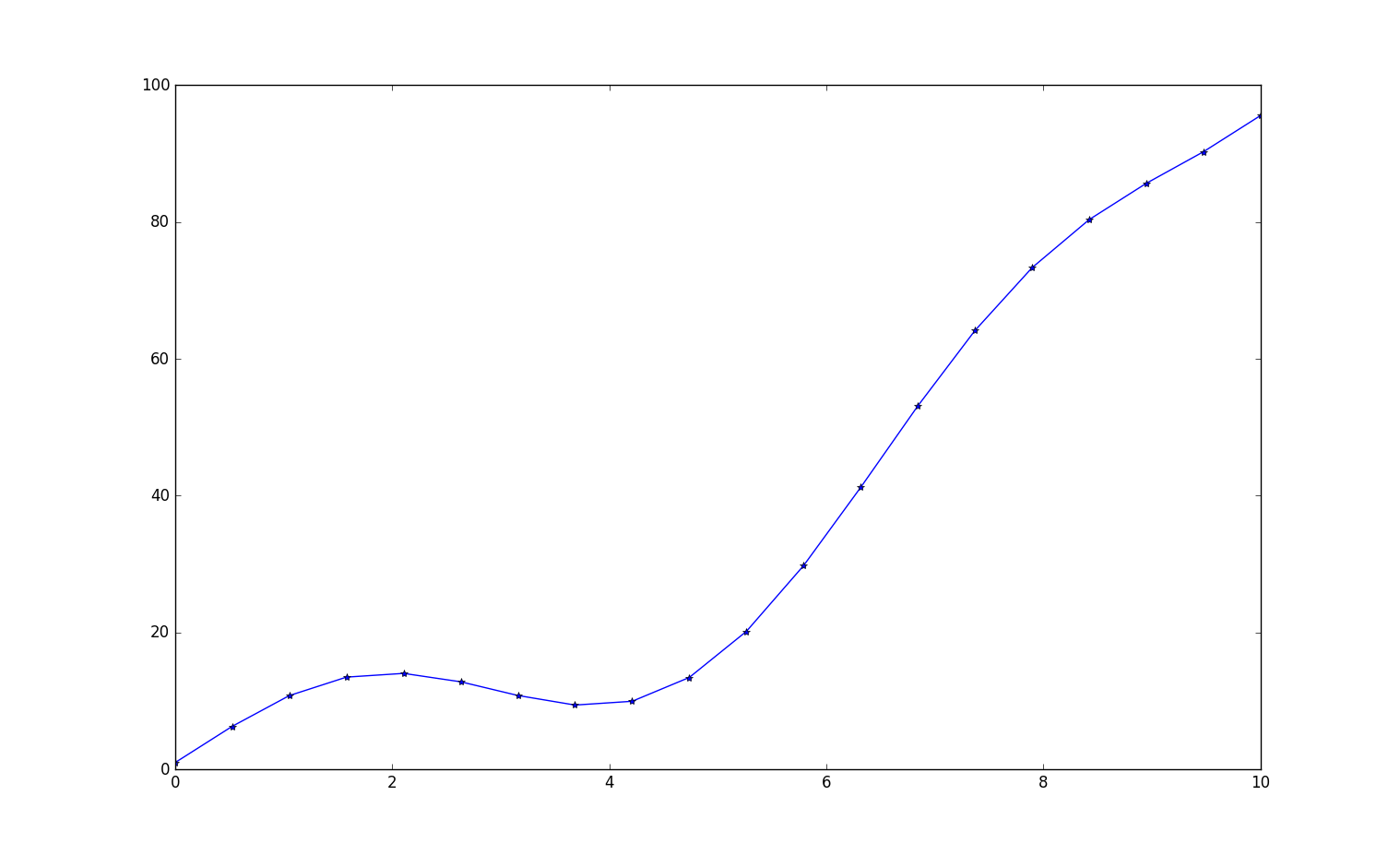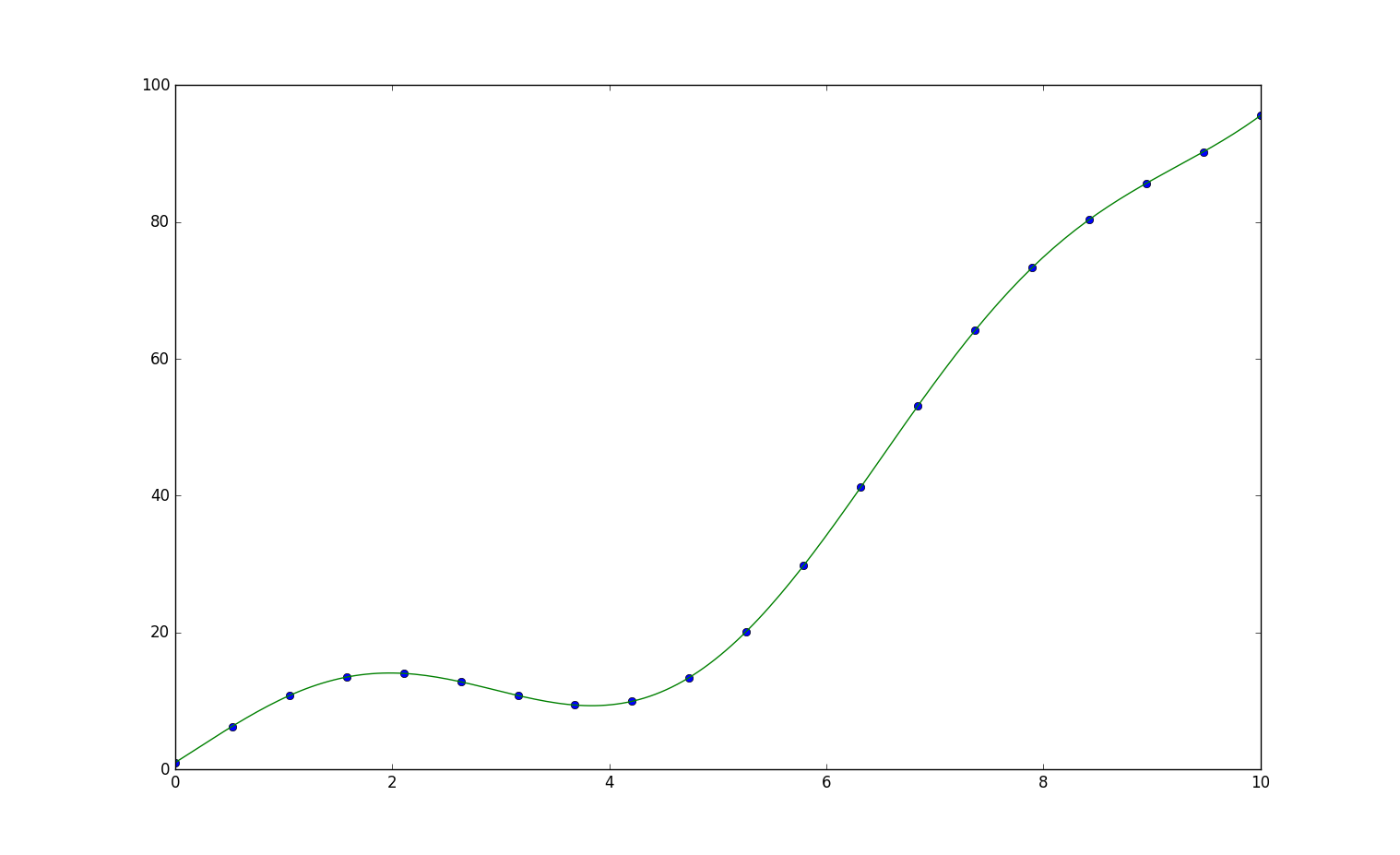# 12. Scipy Tutorial-一元样条插值

scipy.interpolate包里提供了两个函数splev和splrep共同完成(B-样条:贝兹曲线(又称贝塞尔曲线))插值，和之前一元插值一步就能完成不同，样条插值需要两步完成，第一步先用splrep计算出b样条曲线的参数tck，第二步在第一步的基础上用splev计算出各取样点的插值结果。

import numpy as np
import matplotlib.pyplot as plt
from scipy.interpolate import splev, splrep
def f(x):
return x ** 2 + 10 * np.sin(x) + 1
x = np.linspace(0, 10, 20)
y = f(x)
plt.plot(x, y, '*-')
plt.show()
x2 = np.linspace(0, 10, 200)
spl = splrep(x, y, s = 0)
y2 = splev(x2, spl)
plt.plot(x, y, 'o', x2, y2)
plt.show()